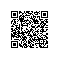# 4、基本结构

(2)对决策过程划分阶段。
(3)对各阶段确定状态变量。
(4)根据状态变量确定费用函数和目标函数。
(5)建立各阶段状态变量的转移过程，确定状态转移方程。状态转移方程的一般形式：一般形式： U：状态； X：策略　顺推
f[Uk]=opt{f[Uk-1]+L[Uk-1,Xk-1]}

# 5、动态规划的基础框架

// 第一个阶段
xn[j] = 初始值;

for(i=n-1; i>=1; i=i-1)
// 其他n-1个阶段
for(j=1; j>=f(i); j=j+1)
//f(i)与i有关的表达式
xi[j]=j=max（或min）{g(xi-1[j1:j2]), ......, g(xi-1[jk:jk+1])};

t = g(x1[j1:j2]);
// 由子问题的最优解求解整个问题的最优解的方案

print(x1[j1]);

for(i=2; i<=n-1; i=i+1）
{
t = t-xi-1[ji];

for(j=1; j>=f(i); j=j+1)
if(t=xi[ji])
break;
}

# 6、动态规划经典问题

01背包问题使用钉钉扫一扫加入圈子
+ 订阅